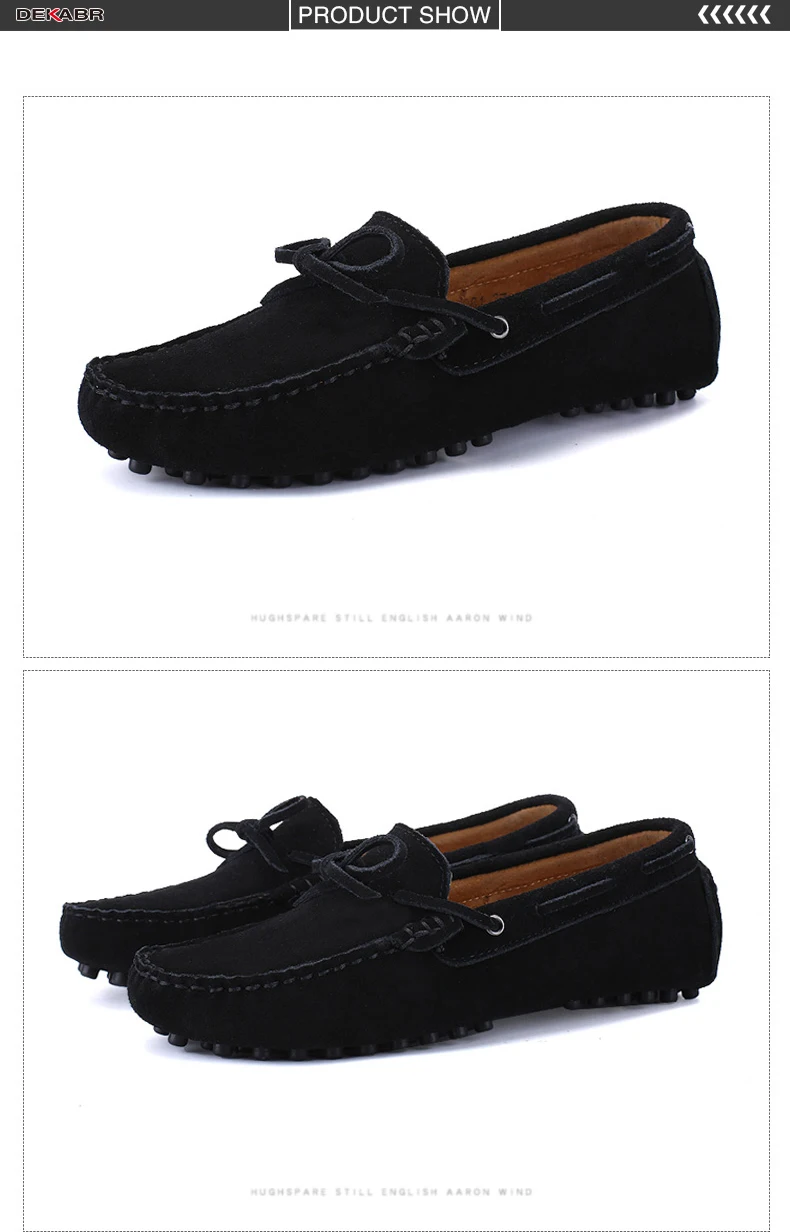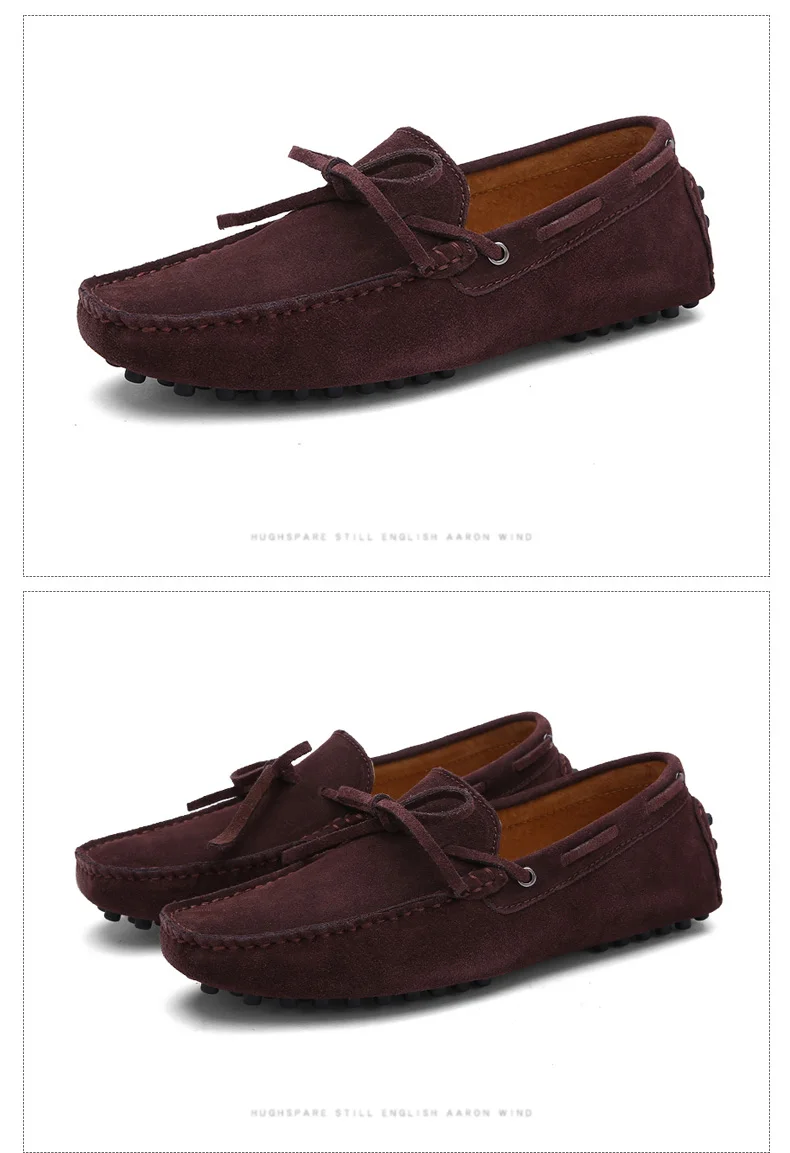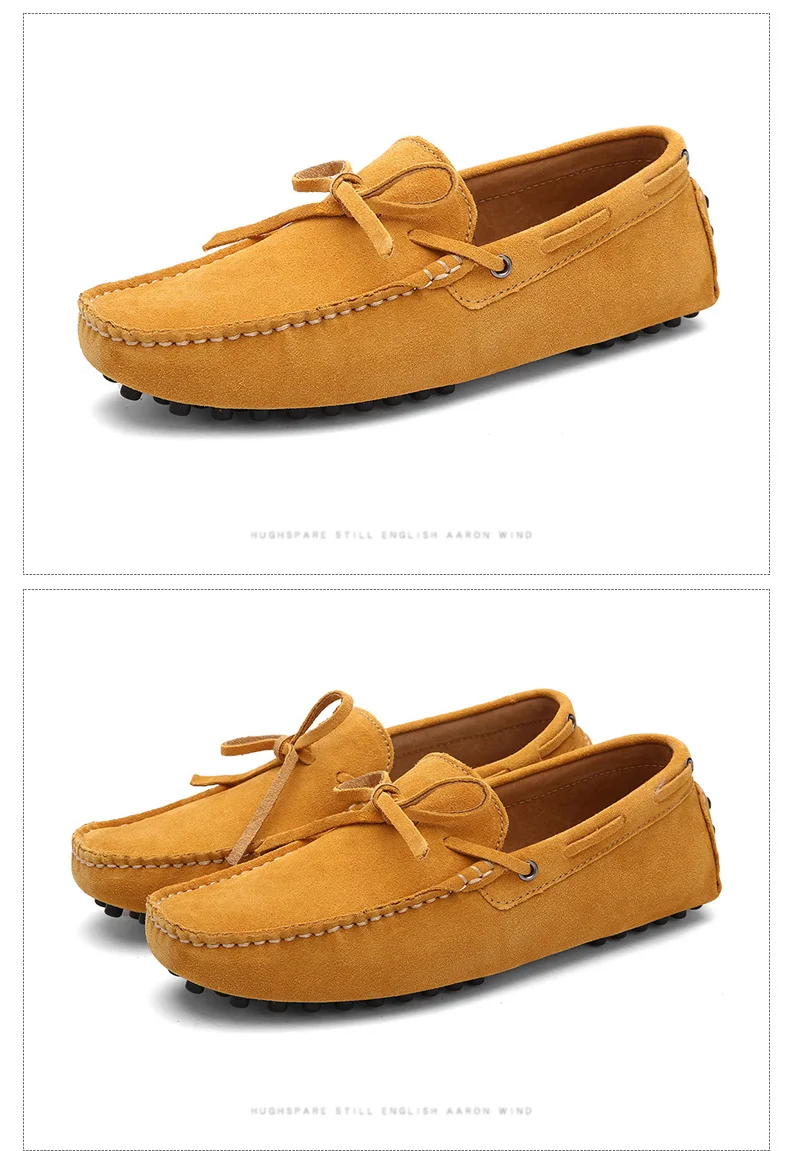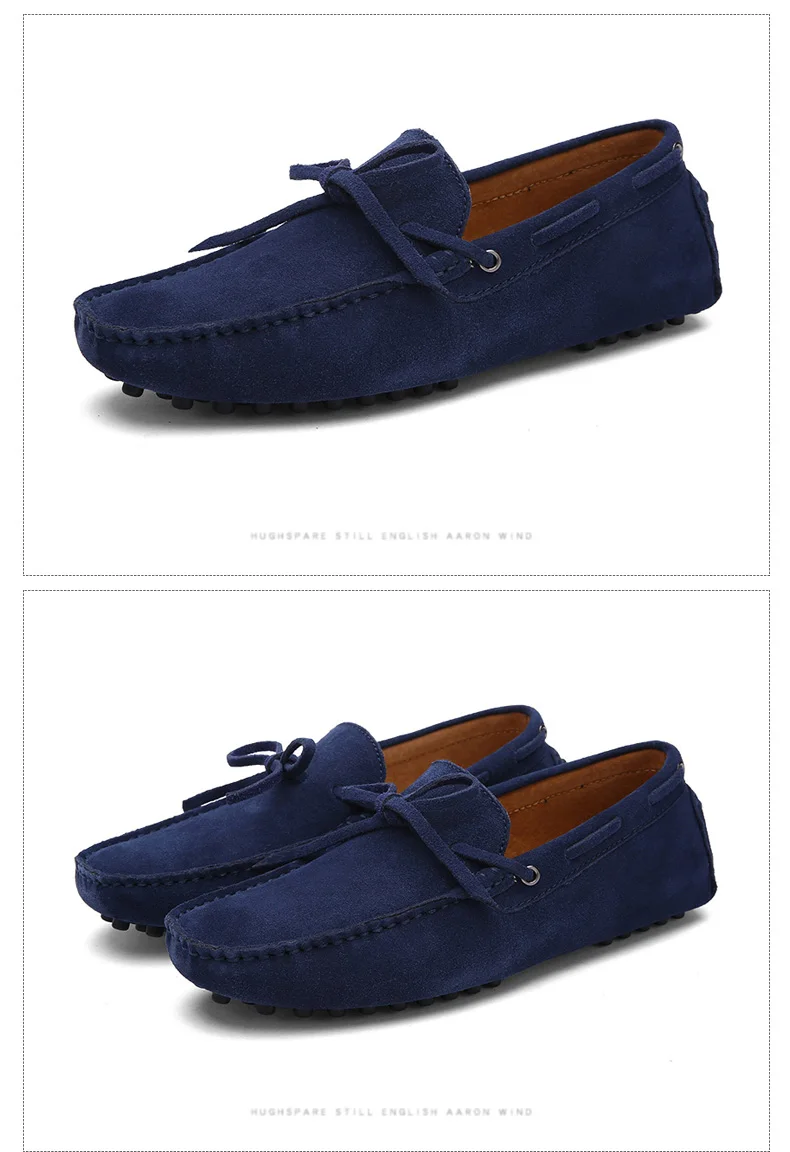# Men Casual Shoes High Quality Men Loafers Moccasin Drivinges

\$58.82
بواسطة HABASH FASHION

• Shoe Type: Boat Shoes
• Upper Material: Cow Suede
• Outsole Material: Rubber
• Closure Type: Slip-On
• Fit: Fits true to size, take your normal size
• Pattern Type: striped
• Feature: Breathable, waterproof
• Insole Material: Bonded Leather
• Lining Material: Genuine Leather
• Big Size: 38,39,40,41,42,43,44,45,46,47,48,49

Hi,Dear value customers! we advising you can choose size according your foot length in our size table.
For example, if your foot length (Heel to Toe) is 26.5 cm,please choose size 8.5=42 in this model.
Size 5=35      fit for foot length is 23cm.
Size 5.5=36   fit for foot length is 23.5cm.
Size 6=37      fit for foot length is 24cm.
Size 6.5=38   fit for foot length is 24.5cm.
Size 7=39      fit for foot length is 25cm.
Size 7.5=40   fit for foot length is 25.5cm.
Size 8=41      fit for foot length is 26cm.
Size 8.5=42   fit for foot length is 26.5cm.
Size 9.5=43   fit for foot length is 27cm.
Size 10=44    fit for foot length is 27.5cm.
Size 11=45    fit for foot length is 28cm
Size 12=46    fit for foot length is 28.5cm.
Size 13=47    fit for foot length is 29cm.
Size 14=48    fit for foot length is 29.5cm.
Size 15=49    fit for foot length is 30cm.